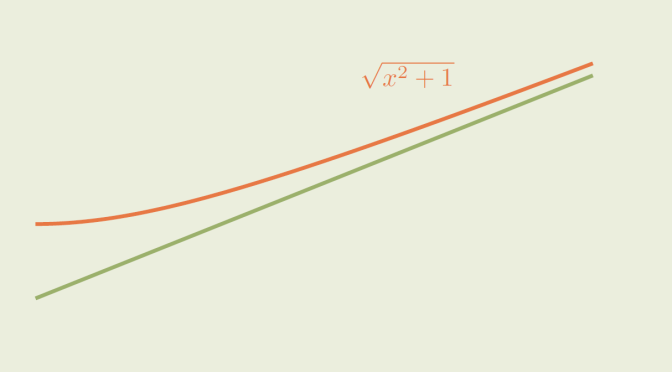# Counterexamples to Banach fixed-point theorem

Let $$(X,d)$$ be a metric space. Then a map $$T : X \to X$$ is called a contraction map if it exists $$0 \le k < 1$$ such that $d(T(x),T(y)) \le k d(x,y)$ for all $$x,y \in X$$. According to Banach fixed-point theorem, if $$(X,d)$$ is a complete metric space and $$T$$ a contraction map, then $$T$$ admits a fixed-point $$x^* \in X$$, i.e. $$T(x^*)=x^*$$.

We look here at counterexamples to the Banach fixed-point theorem when some hypothesis are not fulfilled.

First, let’s consider $\begin{array}{l|rcl} f : & \mathbb R & \longrightarrow & \mathbb R \\ & x & \longmapsto & x+1 \end{array}$ For all $$x,y \in \mathbb R$$ we have $$\vert f(x)-f(y) \vert = \vert x- y \vert$$. $$f$$ is not a contraction, but an isometry. Obviously, $$f$$ has no fixed-point.

We now prove that a map satisfying $d(g(x),g(y)) < d(x,y)$ might also not have a fixed-point. A counterexample is the following map $\begin{array}{l|rcl} g : & [0,+\infty) & \longrightarrow & [0,+\infty) \\ & x & \longmapsto & \sqrt{1+x^2} \end{array}$ Since $g^\prime(\xi) = \frac{\xi}{\sqrt{1+\xi^2}} < 1 \text{ for all } \xi \in [0, +\infty),$ by the mean value theorem $\vert g(x) - g(y)| = \vert g^\prime(\xi)\vert |x-y| < |x-y| \text{ for all } x, y \in [0, +\infty).$ However $$g$$ has no fixed-point. Finally, let's have a look to a space $$(X,d)$$ which is not complete. We take $$a,b \in \mathbb R$$ with $$0 < a < 1$$ and for $$(X,d)$$ the space $$X = \mathbb R \setminus \{\frac{b}{1-a}\}$$ equipped with absolute value distance. $$X$$ is not complete. Consider the map $\begin{array}{l|rcl} h : & X & \longrightarrow & X \\ & x & \longmapsto & ax + b \end{array}$ $$h$$ is well defined as for $$x \neq \frac{b}{1-a}$$, $$h(x) \neq \frac{b}{1-a}$$. $$h$$ is a contraction map as for $$x,y \in \mathbb R$$ $\vert h(x)-h(y) \vert = a \vert x - y \vert$ However, $$h$$ doesn't have a fixed-point in $$X$$ as $$\frac{b}{1-a}$$ is the only real for which $$h(x)=x$$.